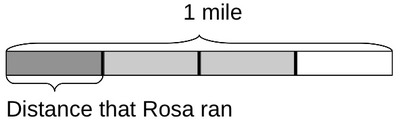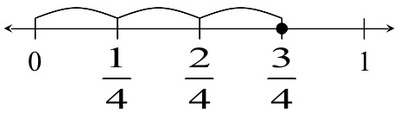# Running to School

Alignments to Content Standards: 5.NF.B.6

The distance between Rosa’s house and her school is $\frac{3}{4}$ mile. She ran $\frac{1}{3}$ of the way to school. How many miles did she run?

## IM Commentary

This is the easiest problem in a series of three tasks involving fraction multiplication that can be solved with pictures or number lines. Students who solve this problem with a picture or using a number line do not need to subdivide the unit fractions that comprise $\frac{3}{4}$ to find $\frac{1}{3}$ of $\frac{3}{4}$ since it is naturally composed of 3 pieces. The next task, 5.NF Drinking Juice, does require students to subdivide the unit fractions that comprise $\frac{1}{2}$ in order to find $\frac{3}{4}$ of $\frac{1}{2}$. The third task, 5.NF Half of a Recipe, also requires subdivision and involves multiplying a fraction and a mixed number.

Since tasks such as this will be among the first that students see, solutions which involve (sub)dividing a quantity into equal parts in order to find a fraction of the quantity should be emphasized. In particular, such solutions should be introduced if students do not generate them on their own. Students benefit from reasoning through the solution to such word problems before they are told that they can be solved by multiplying the fractions; this helps them develop meaning for fraction multiplication.

Also note that the context itself, namely a distance, naturally lends itself to drawing a tape diagram or number line (both models based on length). The next two tasks in this series involve other types of quantities, making the number lines in particular more abstract representations relative to the context.

## Solutions

Solution: Drawing a Picture

The first picture shows that the distance between Rosa’s house and the school is $\frac{3}{4}$ mile.$\frac34$ already has three equal parts, so one of them is $\frac13$ of $\frac34$. In the next picture, $\frac{1}{3}$ of the distance is shaded more darkly.Rosa ran $\frac{1}{4}$ mile.

Solution: Using a Number Line

First plot a point at $\frac{3}{4}$, which represents the distance between home and school.Since $\frac{3}{4}$ is 3 equal jumps away from 0, one jump (or $\frac{1}{4}$) is $\frac{1}{3}$ of $\frac{3}{4}$.So Rosa ran $\frac{1}{4}$ mile.

Solution: Computing a Product

Since we want to know what $\frac{1}{3}$ of $\frac{3}{4}$ miles is, we can multiply: \begin{equation} \frac{1}{3} \times \frac{3}{4} = \frac{1 \times 3}{3 \times 4} = \frac{3}{12} = \frac{1}{4} \end{equation} Rosa ran $\frac{1}{4}$ mile.

Solution: Use Decimal Numbers

A student at this level may call on his or her understanding of decimal numbers and operations, noting that $\frac{3}{4}$ mile $= 0.75$ miles. Dividing by three to obtain one third of $0.75$ miles gives

$$0.75 \mathrm{mi} \div 3 = 0.25 \mathrm{mi}.$$